# Harmonic Numbers and the Book-stacking Problem

An interesting problem is to determine how far an overhang we can achieve by stacking books on a table, accounting for the force of gravity.

To balance one book on a table, the center of gravity of the book must be somewhere over the table; to achieve the maximum overhang, the center of gravity should be just over the table’s edge. Assuming a book is one unit in length (and assuming the book is placed with a steady hand), the maximum overhang we can achieve with one book is 1/2 unit.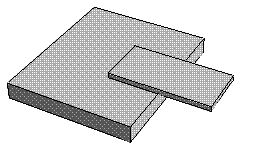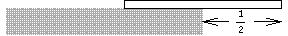For two books, the center of gravity of the first should be directly over the edge of the second, and the center of gravity of the stack of two books should be directly above the edge of the table. The center of gravity of the stack of two books is at the midpoint of the books’ overlap, or (1 + 1/2)/2, which is 3/4 unit from the far end of the top book.

It turns out that the overhangs are related to the harmonic numbers Hn, which are defined as 1 + 1/2 + 1/3 + ... + 1/n: the maximum overhang possible for n books is Hn/2.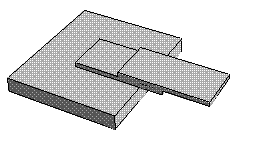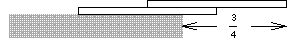With four books, the overhang (1 + 1/2 + 1/3 + 1/4)/2 exceeds 1 (it’s 25/24), so no part of the top book is directly over the table.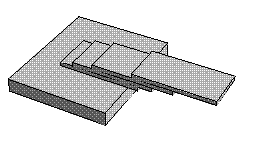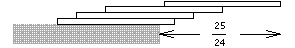With 31 books, we achieve over two book lengths of overhang: 2.01362 book lengths, in fact (30 books give us just 1.99749 lengths’ overhang).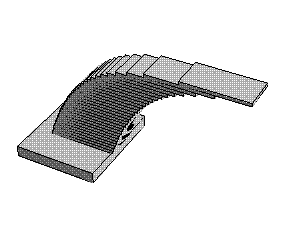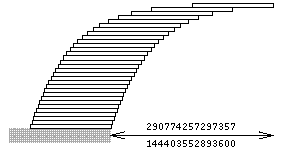Originally designed and rendered long ago with Mathematica 3.0 and GifOMatic for NeXT.